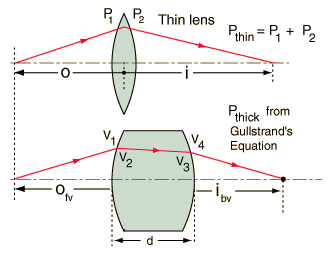# Vergence Tracing in Thick Lens

Thick lenses can be analyzed in terms of vergence. The vergence can be traced by noting its change at each surface.A thick lens can be characterized by its radii of curvature, its index of refraction, and its thickness. For glass with index nlens = the front surface power for lens radius R1 = m is P1 = m-1 and the back surface power for lens radius R2 = m is P2 = m-1

Lens thickness d = cm = m

(Note that for a double convex lens, the front surface radius R1 is positive and the back surface radius R2 is negative according to the Cartesian sign convention.)

The vergences can be calculated from the lens parameters and the object distance.The change in vergence when the light encounters a refracting surface is equal to the power of the surface:

Using the Cartesian sign convention, the object distance is typically a negative number since it points opposite to the direction of light travel.

Object distance o = m.

The vergences can then be calculated.

V1 = n0/o = m-1

V2 = V1 + P1 = n/i1 =m-1

V3 = n/(i1 - d) = m-1

V4 = V3 + P2 = n0/i =m-1

The value i1 calculated as an intermediate value above would be the image distance inside the glass with just the front surface power acting. This calculation presumes that this image distance is greater than the thickness of the lens. It is also presumed that the medium is air so that n0 = 1.

The exit vergence from the final surface determines the image distance with respect to that surface.

Image distance i = n0/V4 = m.

This can be compared with the image distance for a thin lens with the same surface powers:

Image distance ithin = m.

The power of a thin lens is just the sum of the surface powers:

Pthin = P1 + P2 = m-1.

The equivalent power for the thick lens can be calculated from Gullstrand's equation:

Pthick = P1 + P2 - P1P2d/n = m-1.

Note that the calculation does not take into account the change in lens thickness with the angle of the incoming ray. It is typical to do the calculation only for the paraxial rays where the departure from full thickness is negligible.

 Compare to same calculation by: Principal plane method
 Vergence Example
 Thick lens in different media
Index

Lens concepts

Thick lens concepts

 HyperPhysics***** Light and Vision R Nave
Go Back